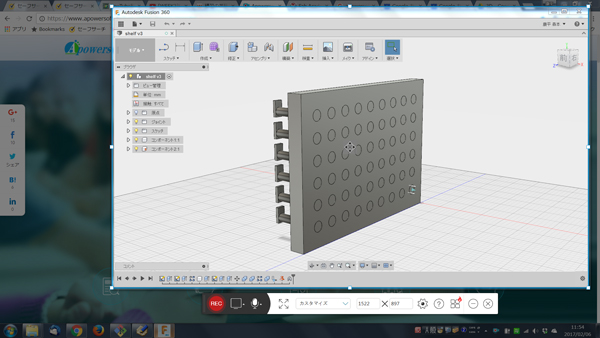## [WEEK2] Computer-Aided Design

### 1. 3D design

#### 1.1 Fusion 360.

I modelled 3D objects with Fusion 360.
This is a prototype for the final project. I won't print all the parts with 3D printer. But I made this as a training and simulation.

Some rods come out from a board coresponding to the input data like a movie below.
I move the components using function of Fusion360.
-set "slide joint"

Each holes are parametorically created. So I can easily change its number or size depending on the specification of the control system.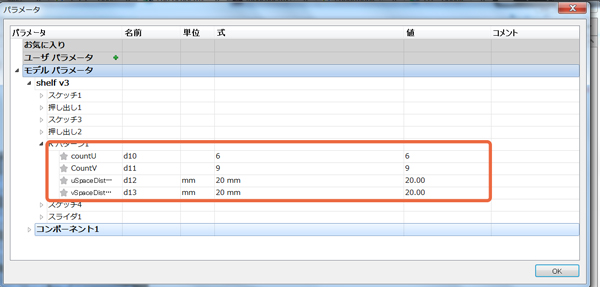This is a rendering image.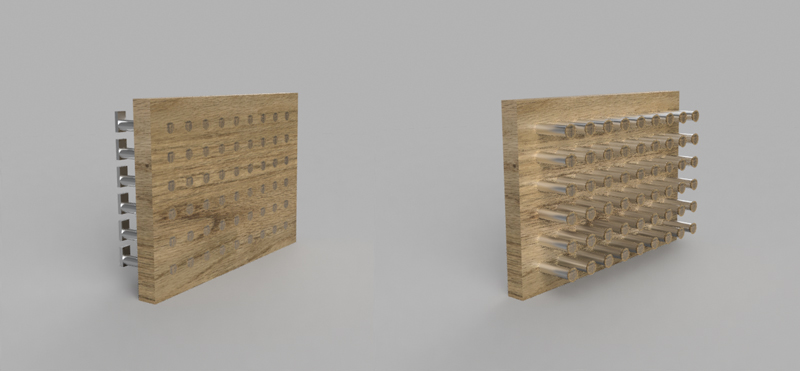After that,I wanted to do strength analysis using the function of fusion 360. I tried it but I couldn’t, because I couldn’t set the condition properly.I continue to study it.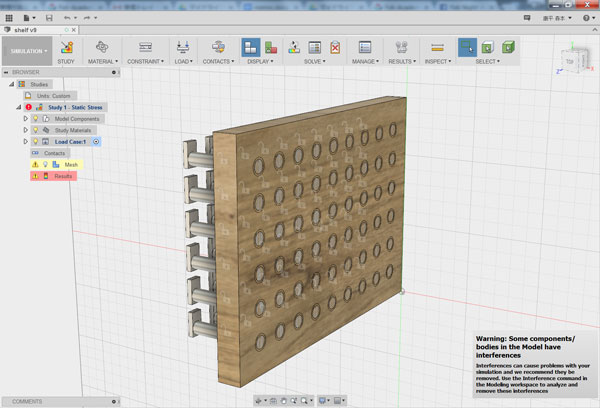I practiced modeling 3D objects with OpenSCAD.
I modelled a tape cutter.(I have made this model with 123D Design before. I simplified it for the exercise.)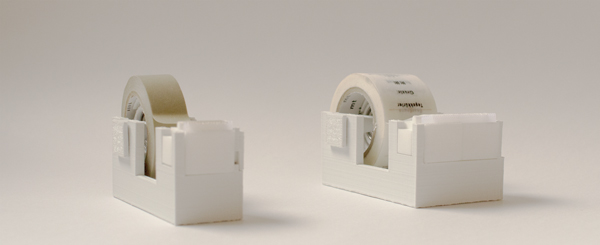It includes three compornents.I aimed to change the size of them in linkage with each other.

1.2.1 Direct modeling

At first, I inputted the values on the files directly.

• Base of Tape cutter
•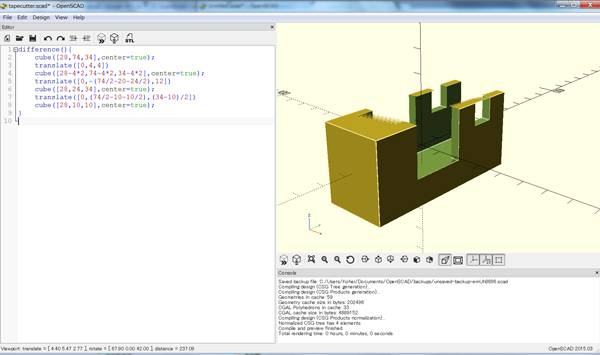```	difference(){
cube([28,74,34],center=true);
translate([0,4,4])
cube([28-4*2,74-4*2,34-4*2],center=true);
translate([0,-(74/2-20-24/2),12])
cube([28,24,34],center=true);
translate([0,(74/2-10-10/2),(34-10)/2])
cube([28,10,10],center=true);
}```
• Bearing holder
•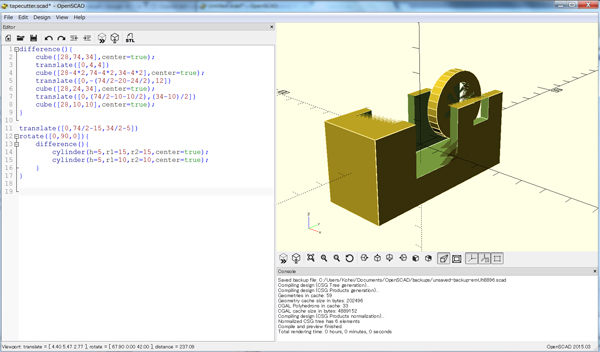```	translate([0,74/2-15,34/2-5])
rotate([0,90,0]){
difference(){
cylinder(h=5,r1=15,r2=15,center=true);
cylinder(h=5,r1=10,r2=10,center=true);
}
}```
• Bearing shaft
•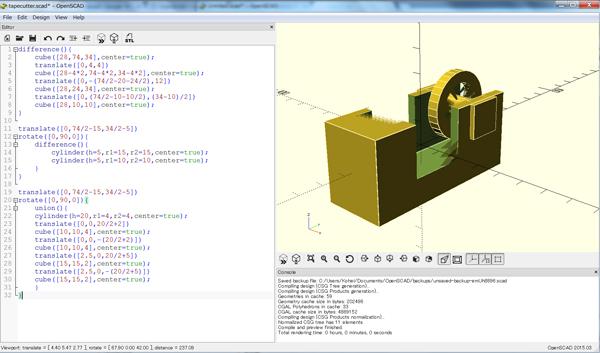```	translate([0,74/2-15,34/2-5])
rotate([0,90,0]){
union(){
cylinder(h=20,r1=4,r2=4,center=true);
translate([0,0,20/2+2])
cube([10,10,4],center=true);
translate([0,0,-(20/2+2)])
cube([10,10,4],center=true);
translate([2.5,0,20/2+5])
cube([15,15,2],center=true);
translate([2.5,0,-(20/2+5)])
cube([15,15,2],center=true);
}
}```

1.2.2 Modularization

In the next step, I converted from the values to the variables and build modules for each components.

• Module of tapecutter base:
• ```	module tapebase (width,depth,height,thickness){
difference(){
cube([width,depth,height],center=true);
translate([0,thickness,thickness])
cube([width-thickness*2,depth-thickness*2,height-thickness*2],center=true);
translate([0,-(depth/2-20-height/2),12])
cube([width,24,height],center=true);
translate([0,(depth/2-10-10/2),(height-10)/2])
cube([width,10,10],center=true);
}
}
```
• Module of bearing holder:
• ```	module bearingholder(width,depth,height,br_outer,br_width,holer_thickness){
translate([0,depth/2-15,height/2-5])
rotate([0,90,0]){
difference(){
cylinder(h=br_width,r1=br_outer+holer_thickness,r2=br_outer+holer_thickness,center=true);
cylinder(h=br_width,r1=br_outer,r2=br_outer,center=true);
}
}
}
```
• Module of bearing shaft:
• ```	module bearingshaft(width,depth,height,thickness,br_inner){
translate([0,depth/2-15,height/2-5])
rotate([0,90,0]){
union(){
cylinder(h=width-thickness*2,r1=br_inner,r2=br_inner,center=true);

translate([0,0,(width-thickness*2)/2+thickness/2])
cube([10,10,thickness],center=true);
translate([0,0,-((width-thickness*2)/2+thickness/2)])
cube([10,10,thickness],center=true);
translate([2.5,0,(width-thickness*2)/2+5])
cube([15,15,2],center=true);
translate([2.5,0,-((width-thickness*2)/2+5)])
cube([15,15,2],center=true);
}
}
}
```
• Modeling with modules:
• ```	tapebase(28,74,34,4);
bearingholder(28,74,34,15,5,5);
bearingshaft(28,74,34,4,4);

translate([60,0,0])
tapebase(48,74,34,4);
translate([60,0,0])
bearingholder(48,74,34,15,10,5);
translate([60,0,0])
bearingshaft(48,74,34,4,4);
```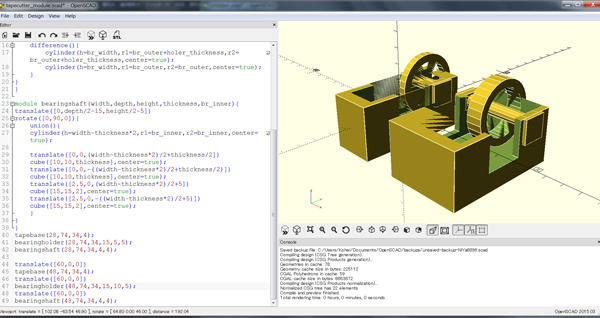I succeeded to model final objects using modules.
But,I set the same variables every modules. I want to set global variables or parents and children function. I don’t understand how to do it. So I will continue to study this software.

### 2. 2D design

I modelled 2D sketches for 2 products.

The one is a box to store the materials and the other is a compornents for the weight measuring instrument.

#### -Image sketch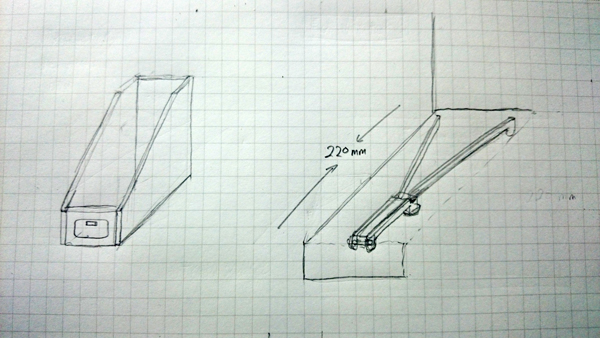I tried to sketch with GIMP.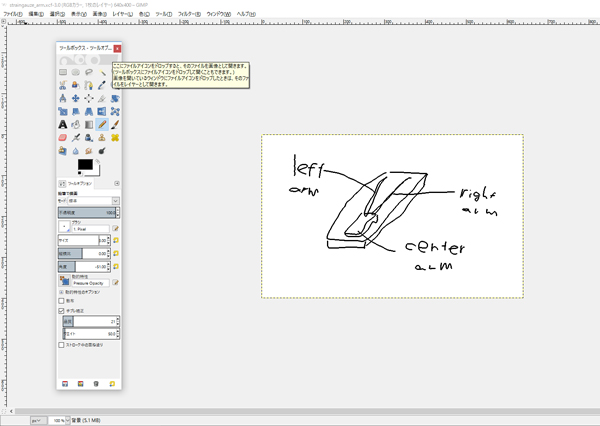But it was a little unreasonable. I want a stylus pen.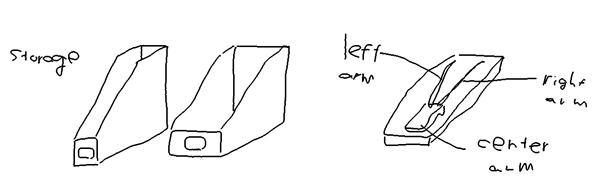#### -Box

The box for sotring materials.I sketched this with Illustrator.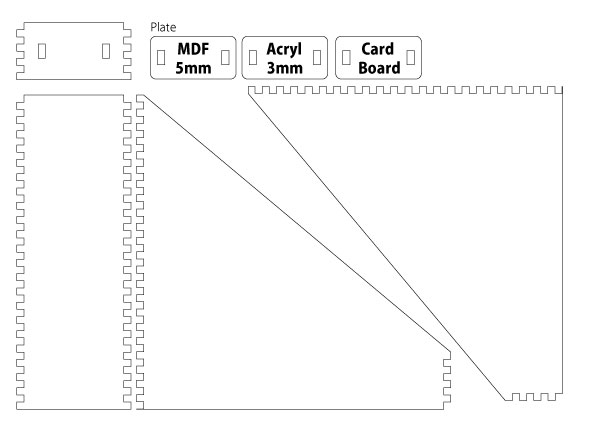#### -Components of the the weight measuring instrument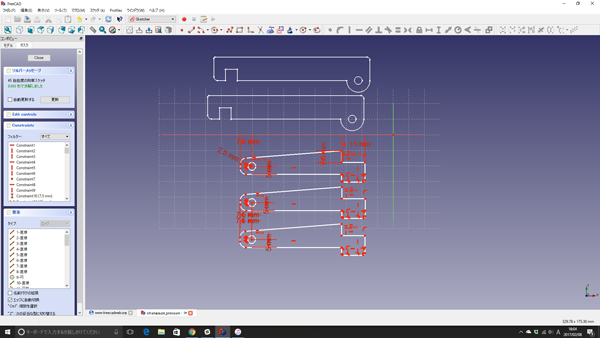FreeCAD is much convenient for sketching mechanical components than graphic software like a Illustrator. I can do almost the same thing by using Fusion 360. But,unlike it, FreeCAD can manage the history of sketching operation. Fusion 360 can do only 3D modeling operation. In addition,FreeCAD supports more formats. These are nice points.